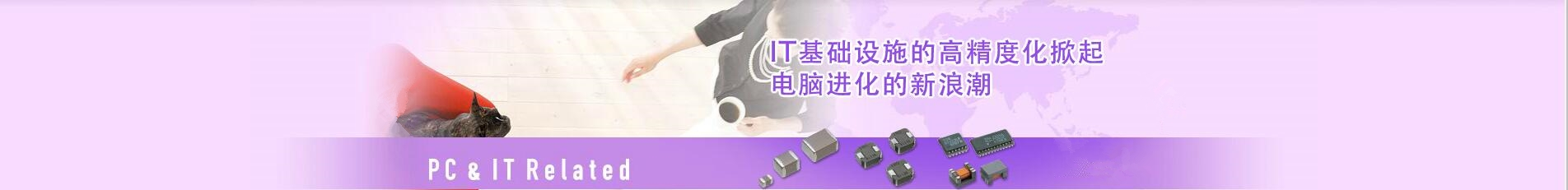## 日本TDK村田murata一级代理商-提供贴片电容器_电感器_蜂鸣器_滤波器_电阻磁珠_电源等产品代理服务

### 深圳市智成电子有限公司

Shenzhen Zhicheng Electronic Co., Ltd.

## 0755-23282269# 详细介绍三星贴片电容相关知识

## 三星贴片电容识别及型号

CL 03 B 104 K Q 8 N N N C
1 2 3 4 5 6 7 8 9 10 11
1 、系列编码：
CL=积层陶瓷电容
2 、尺寸编码
03=0201（0603） 21=0805（2012） 42=1808（4520）
05=0402（1005） 31=1206（3216） 43=1812（4532）
10=0603（1608） 32=1210（3225） 55=2220（5750）
14=0504（1410） 01=0306（0816） 12=0508（1220）
3 、介质
I类 II类
C=C0G S=S2H L=S2L
P=P2H T=T2H
R=R2H U=U2J
A=X5R F=Y5V
B=X7R X=X6S
4 、容量

5 、电容的误差：
B=±0.1pf F=±1pf±1% K=±10%
C=±0.25pf G=±2% M=±20%
D=±0.5pf？ J=±5% Z=+80/-20%
6 、额定电压
R=4V O =16V B =50V E = 250V I = 1000V
Q=6.3V A =25V C=100V G = 500V J = 2000V
P =10V L =35V D =200V H = 630V K= 3000V
7 、厚度：
3=0.30毫米 A=0.65毫米 M=1.15毫米 I=2.00毫米 Q=1.25毫米
5=0.50毫米 C=0.85毫米 F=1.25毫米 J=2.50毫米 V=2.50毫米
8=0.80毫米 D=1.00毫米 H=1.60毫米 L=3.20毫米
8 、内电极
A=常规产品 钯/银/镍屏蔽/锡 100%
N=常规产品 镍/铜/镍屏蔽/锡 100%
G=常规产品 铜/铜/镍屏蔽/锡 100%
L=低侧面产品 镍/铜/镍屏蔽/锡 100%
9、 产品编码
A =阵列（2-元素） L =LICC
B =阵列（4-元素） N =常规
P =自动 C=高频
10、 特殊编码
11 、包装编码
B=散装 O=纸版箱料带，10英寸料盘 E=压花纸版箱，7英寸料盘
P=散装箱 D=纸版箱料带，13英寸料盘（10000ea） F=压花纸版箱，13英寸料盘
C=纸版箱料带，7英寸料盘 L=纸版箱料带，13英寸料盘（15，000ea） S=压花纸版箱，10英寸料盘

## 三星贴片电容包装编码标签识别

①三星贴片电容的编带粘胶都是统一是白色和一小截蓝色；

②拉开编带大约10-12公分处，会有一颗单独的测试料，只有三星才会有的；

③由于喷墨的设置原因，上方标签用手摸起来会有凹凸不平的感觉，比较有质感；

④三星电容的籽颜色会偏淡一些，相比较国巨的货，颜色偏黄，对比起来很明显；

⑤三星贴片电容的盘子比较透明不是纯白，标签处会比较窄一些，大约刚好够放一张标的位置；

⑥原产地：东莞、天津、苏州，商标在字样底部设置精细白点作防伪标记，图像清晰，布面密集，横纵距离一致，并没有其它模糊图像或条纹现象。

## 三星电容的批次号怎么看

1、年Y代表2012，Z代表2013，A代表2014，B代表2015，下一年依次类推。

2、月份1~9，A代表10月，B代表11月,C代表12月

3、日期1~9，A代表10号,B代表11号……依次类推

A=自动化编码;D=年份;8=月份;Q=日份;O=产地;DF=投产日期序号、那么这就是2017年8月26日，年份字母代表有：X=2011年 Y=2012年 Z=2013年 A=2014年 B=2015年。

月份字母代表有：1=1月 2=2月 3=3月 4=4月以此类推到9月 A=10月 B=11月 C=12月。日份字母代表有：1=1日 2=2日 3=3日 4=4日 5=5日 6=6日 7=7日 8=8日9=9日 A=10日 B=11日 C=12日 以此类推。

产地字母代表有：K=(Korea HQ)韩国总部;M=(Mrxico)墨西哥;B=(Korea Busan)韩国釜山厂;O=(Dsem)东莞厂;P=(Philipping)菲律宾厂;S=(Soochow)苏州厂;T I J (Tsem)天津厂;T=(表示全程于天津厂制造);I=(表示抛光制造后于天津厂制造;J=(表示谨于天津厂)。

0755-23282269